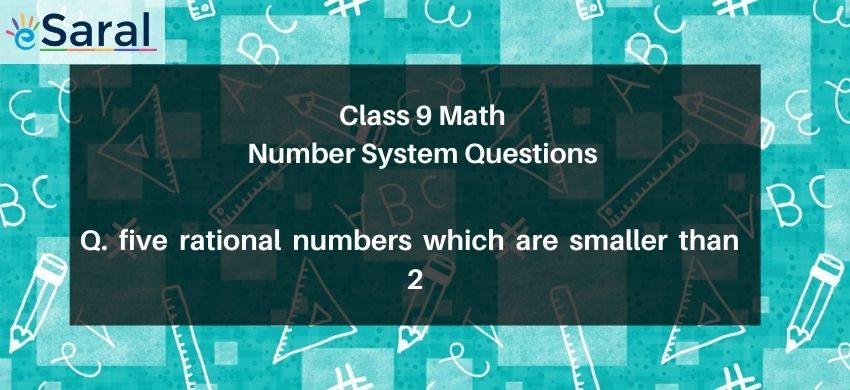Download the eSaral app and start learning from Kota's top IITians and doctors.

# five rational numbers which are smaller than 2# Question : Following are the five rational numbers that are smaller than 2 $\Rightarrow 1, \frac{1}{2}, 0,-1, \frac{-1}{2}$If true then enter 1 and if false then enter 0

Solution :
Correct option is 1
Any number in the form of $\frac{p}{q}$ which is less than 2 will form the answer.
So given numbers are $1, \frac{1}{2}, 0,-1, \frac{-1}{2}$ rational number which are smaller than 2
So the statement is true.